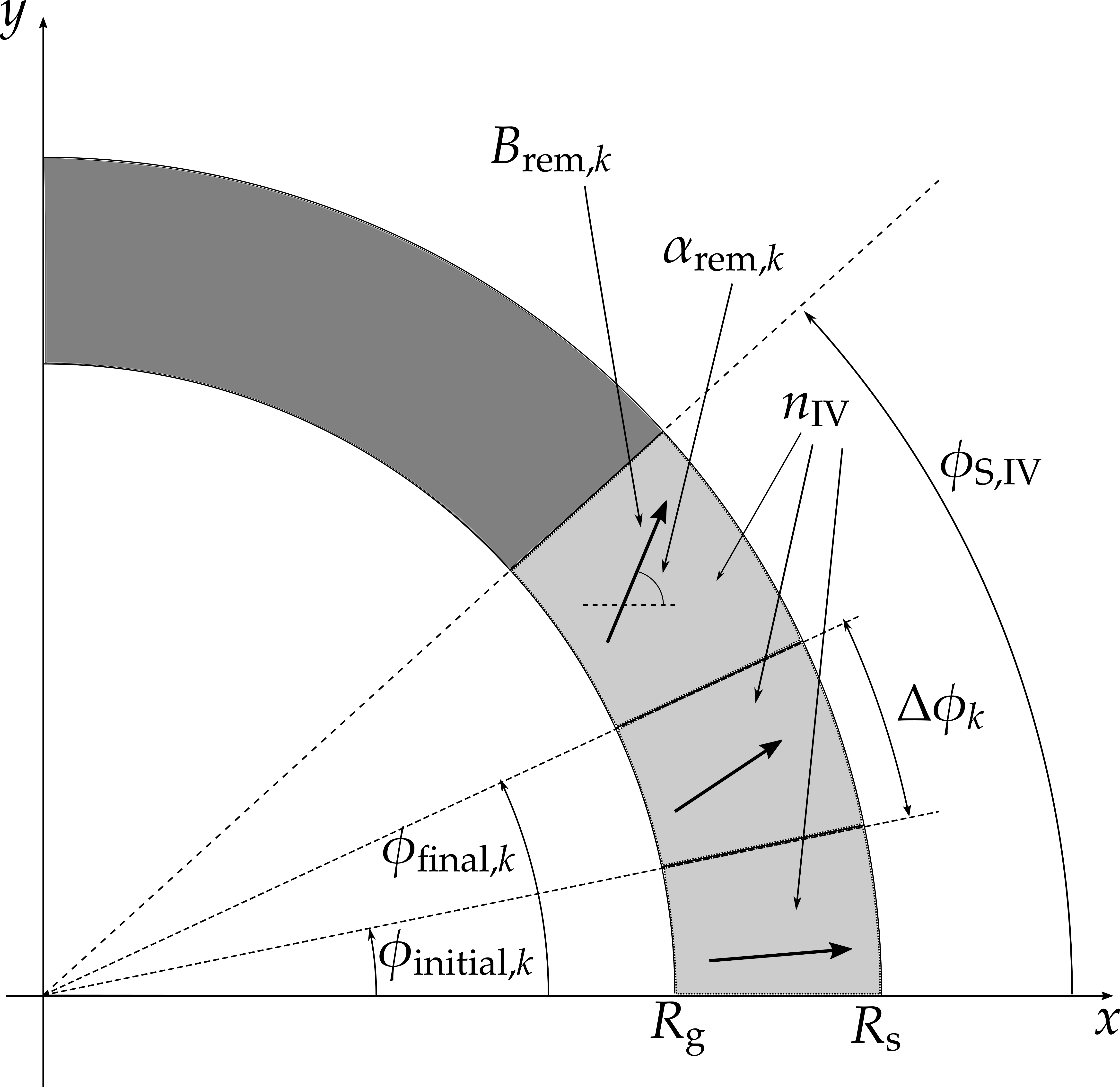{{ message }}

Instantly share code, notes, and snippets.

###fabiofortkamp commented Oct 7, 2019
 This snippet corresponds to the problem of finding the remanence angles following this schematic figure:There are $n_{\mathrm{IV}}$ wedge-like segments, each with its inital and final angular coordinates; each segment is represented by the index $k$. Their "angular widths" $\Delta \phi_k$ are equivalent to the differences between final and initial angular coordinates. The code calculates the angular coordinates of the center point of each segment.